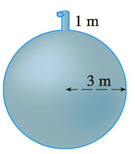Chapter 5.4, Problem 24E

Chapter
Section
Textbook Problem

# A tank is full of water. Find the work required to pump the water out of the spout. In Exercises 25 and 26 use the fact that water weighs 62.5  lb/ft 3 .To determine

To find:

The work required to pump the water out of the spout.

Explanation

1) Concept:

The work is calculated by using the formula W=abf(x)dx

weight=gravity·volume

Area of circular disk is πr2m3

2) Given:

3) Calculation:

From the given figure observe that the given tank is spherical in shape.

The radius of the tank below the center is -3m and from the tap to the center, the height is -4m.Let centre be orgin and let the vertical down the centre and passing through the north and south pole of sphere be x-axis. The x-axis is positive downwards.

Consider circular disk slice of water having thickness x m  lying at the coordinate x. Its’ radius r=9-x2

Volume of the disk of water  =πr2 x m3=π9-x22x m3=π9-x2x m3

Therefore, the weight of the slice is =g.density·volume=9.8×103π9-x2x  N

This weight is lifted by the pump to about x+4m height.s

Therefore, the work needed to pump the slice water out of the spout is

=9.8×103x+4π9-x2x J

Therefore, the total work reqiured is

W=abf(x)dx

W-339.8×103πx+49-x2dx

=9

### Still sussing out bartleby?

Check out a sample textbook solution.

See a sample solution

#### The Solution to Your Study Problems

Bartleby provides explanations to thousands of textbook problems written by our experts, many with advanced degrees!

Get Started

#### Find more solutions based on key concepts